# Volume Of Right Circular Cylinder Lesson Plan For Mathematics Class 9th And 10thHello Friends, Welcome To Our Website. How Are You? Hope You Are Doing Well,

If You Are Searching For Volume Of Right Circular Cylinder Lesson Plan Then You Have Come To The Right Place. Here We Have Shared The Lesson Plan On Volume Of Right Circular Cylinder For B.Ed, D.El.Ed, And School Teachers.

This Mega And Real School Teaching Mathematics Lesson Plan On Volume Of Right Circular Cylinder Is Specially Made For The B.Ed 1st And 2nd Year Students But All The Trainee Teacher And School Teachers Of All Classes Can Prepare Their Daily Teaching Plan Very Easily With The Help Of This Model Right Circular Cylinder Volume Lesson Plan For Class 9 - 12.

Brief Overview Of The Lesson Plan

 Class 9th, 10th Topic Volume Of Right Circular Cylinder Subject Math Lesson Plan Type Mega And Real School Teaching Skill School Teaching

The Topics And Points That Are Covered In This Math Lesson Plan On Volume Of Right Circular Cylinder Are : Introduction To Volume, Formula Of Volume Of Right Circular Cylinder, Questions-Based On Right Circular Cylinder, Volume Of Cylinder Radius And Circumference with Suitable Examples And Homework…..

## Volume Of Right Circular Cylinder Lesson Plan –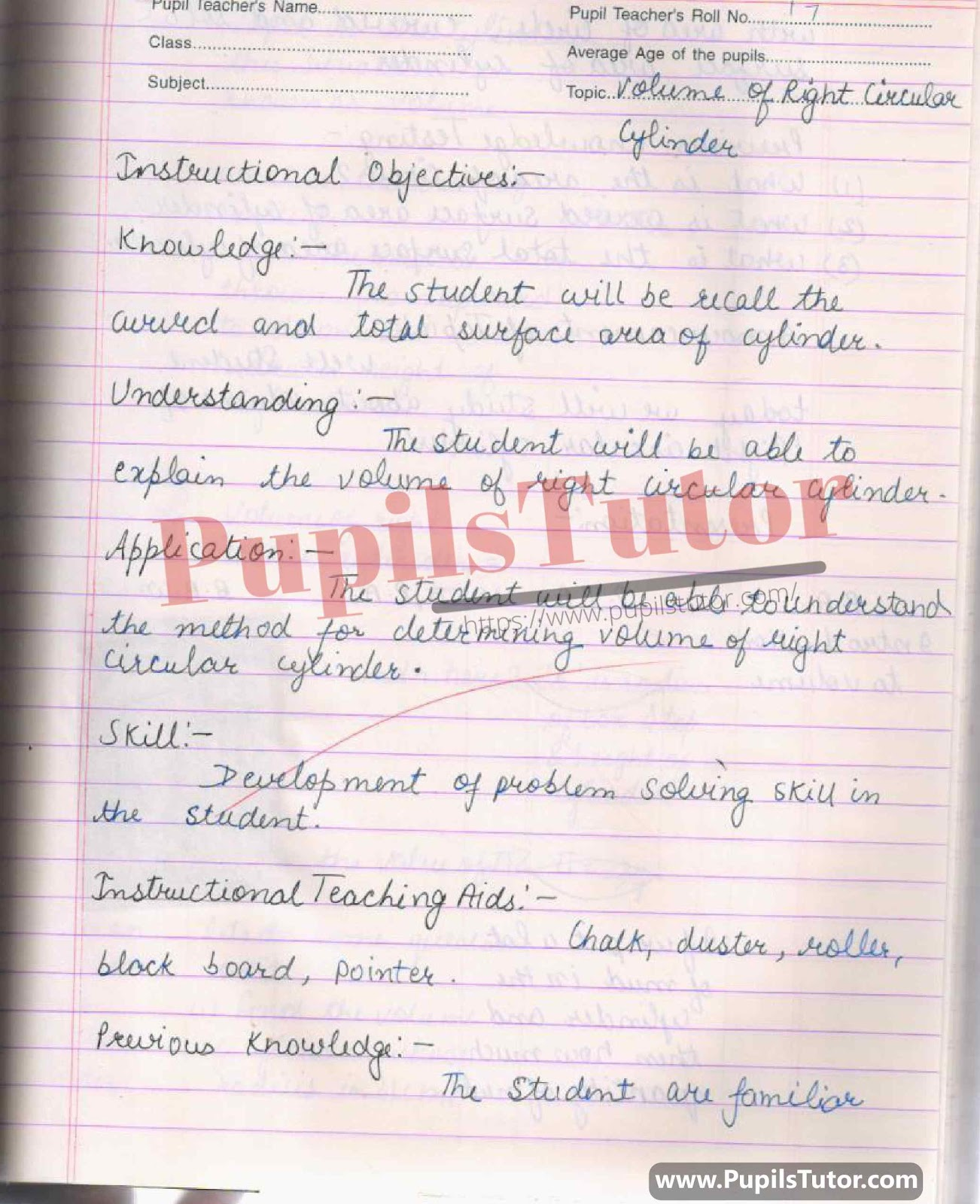## Latest Real School Teaching And Practice Mathematics Lesson Plan On Cylinder, Right Circular Cylinder, Volume Of Right Circular Cylinder For B.Ed And Deled In English Free Download PDF And PPT  –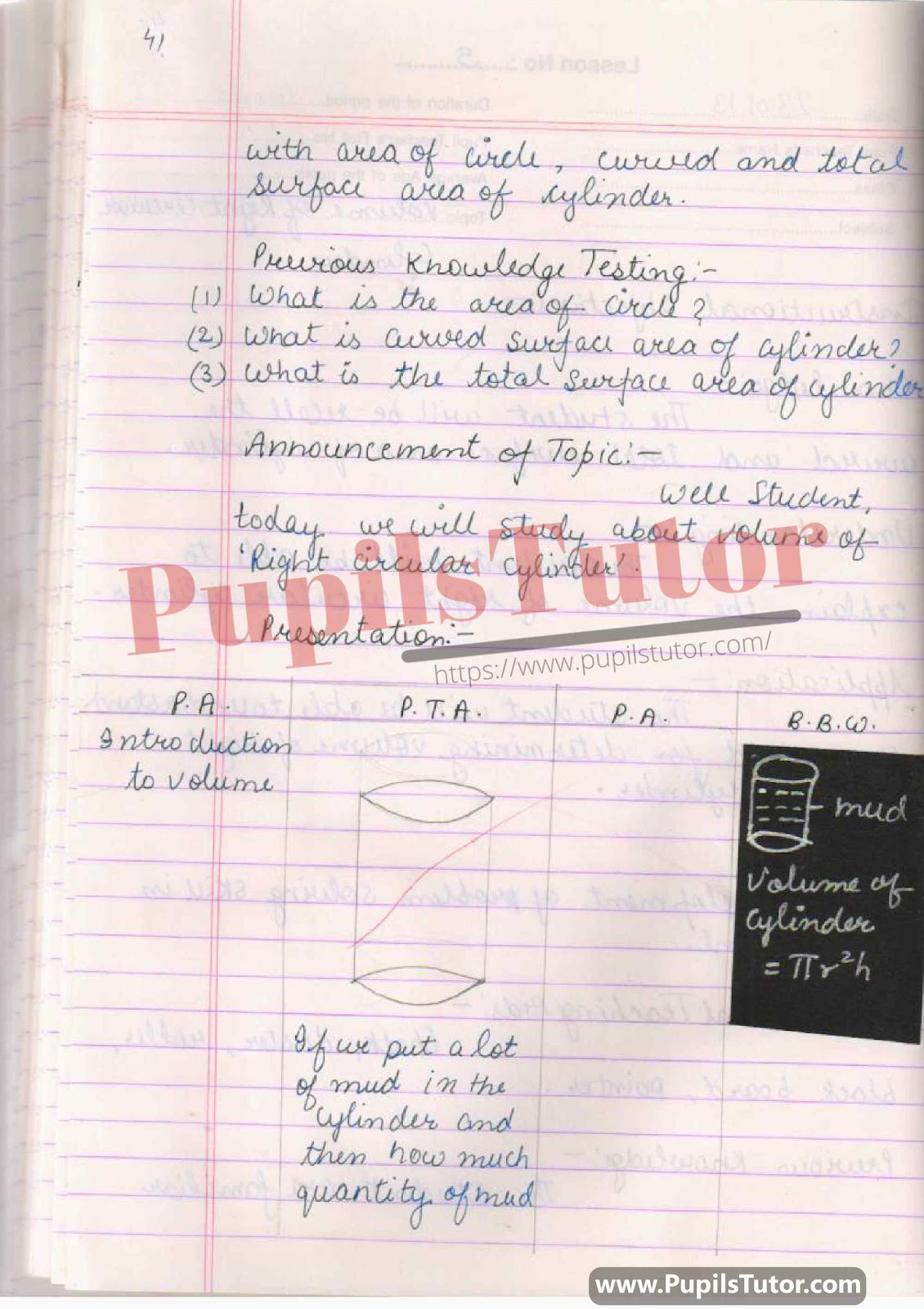## Mathematics Lesson Plan On What Is Volume, Area Of Circle, Radius(r), Height(h), Volume Of Right Circular Cylinder Formula, Questions Based On Volume Of Right Circular Cylinder For Class/Grade 10 For CBSE NCERT School And College Teachers –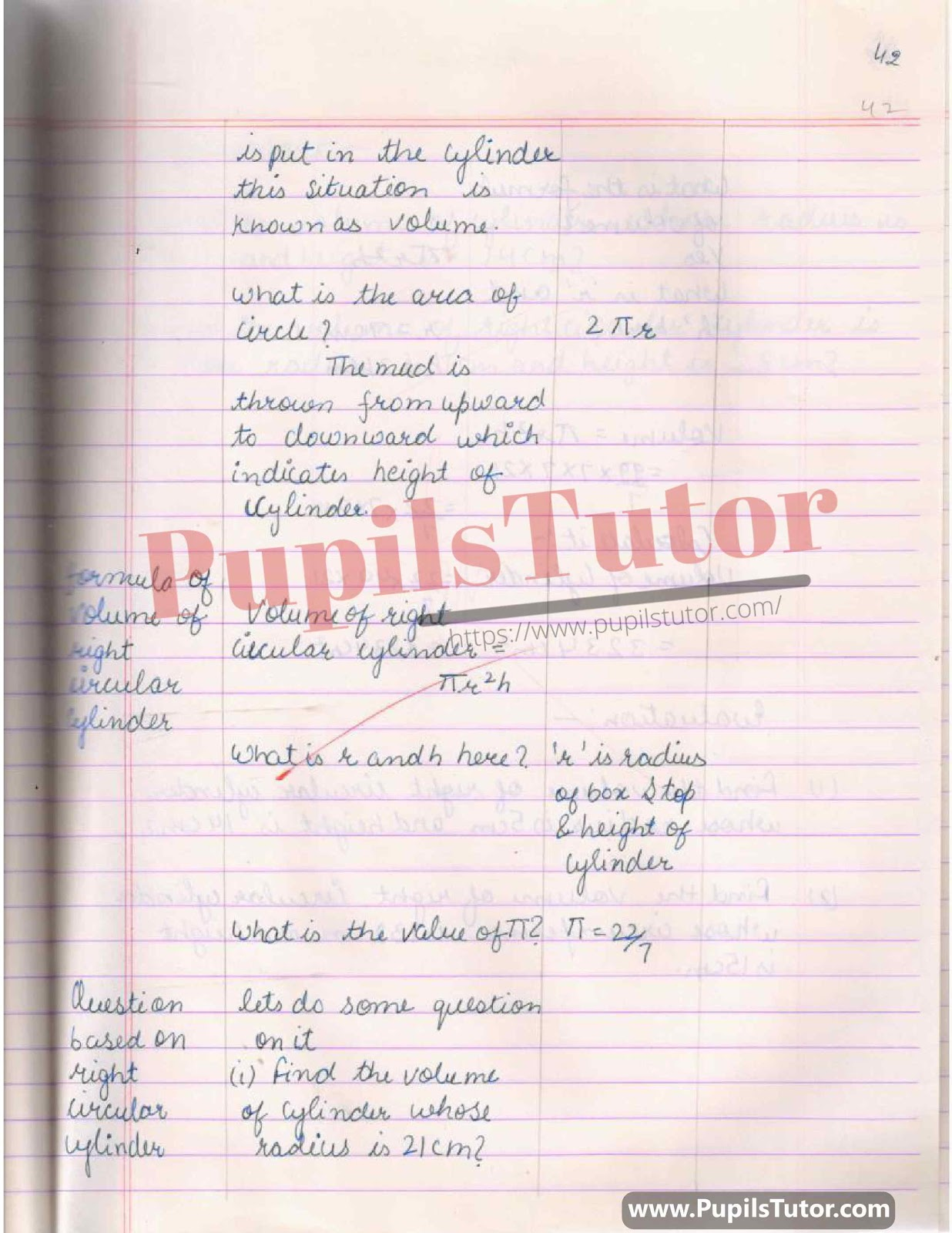## BED, DELED, BTC, BSTC, M.ED, DED And NIOS Teaching Of Math Innovative Digital Lesson Plan Format On Volume Of Cylinder, Cylinder, Geometric Shapes, Volume Of Right Circular Cylinder Topic For Class 6th 7th 8th 9th, 10th, 11th, 12th –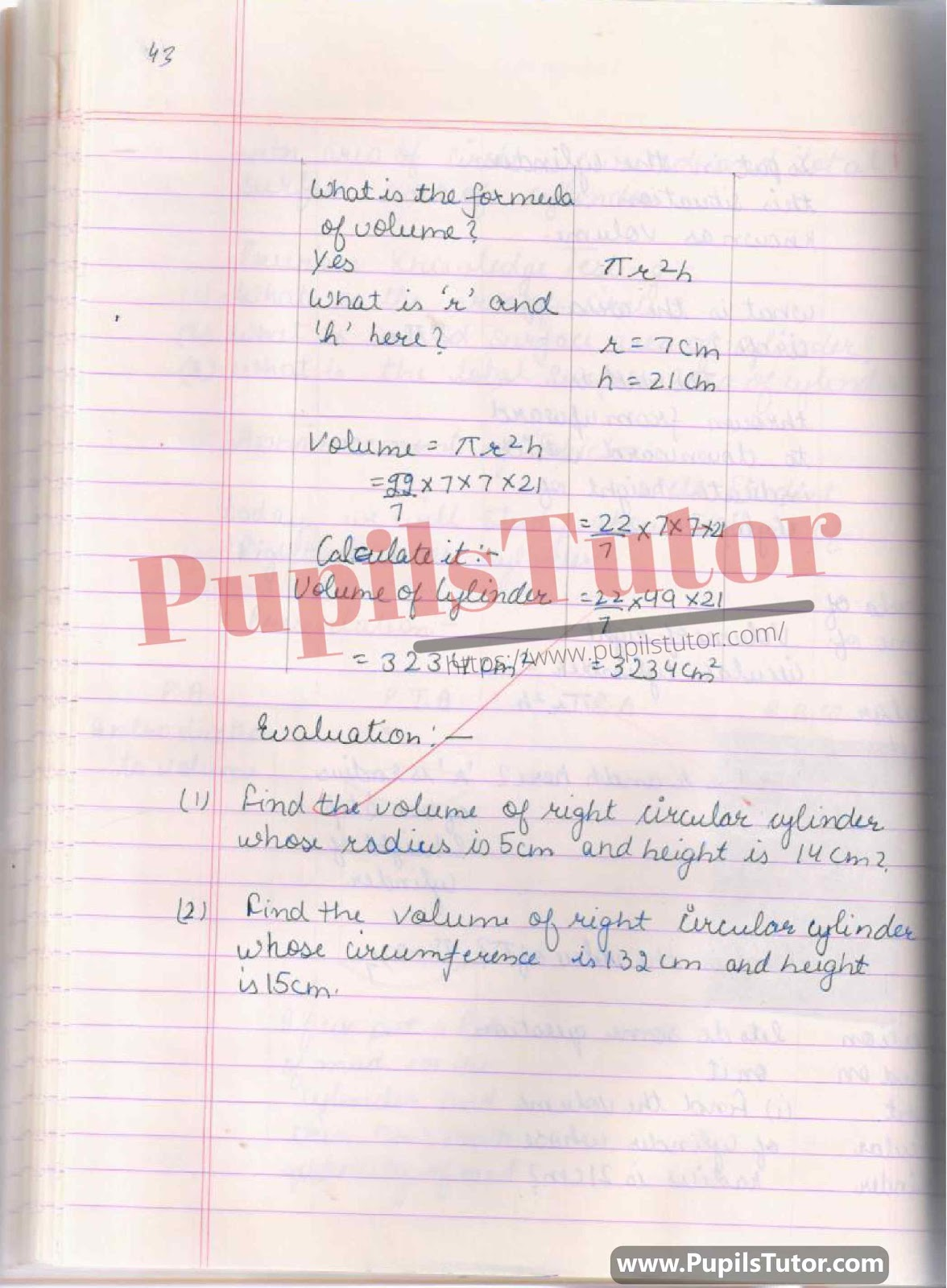## Maths Lesson Plan On Volume Of Right Circular Cylinder(Volume Of Cylinder) For Class 9th And 10th. –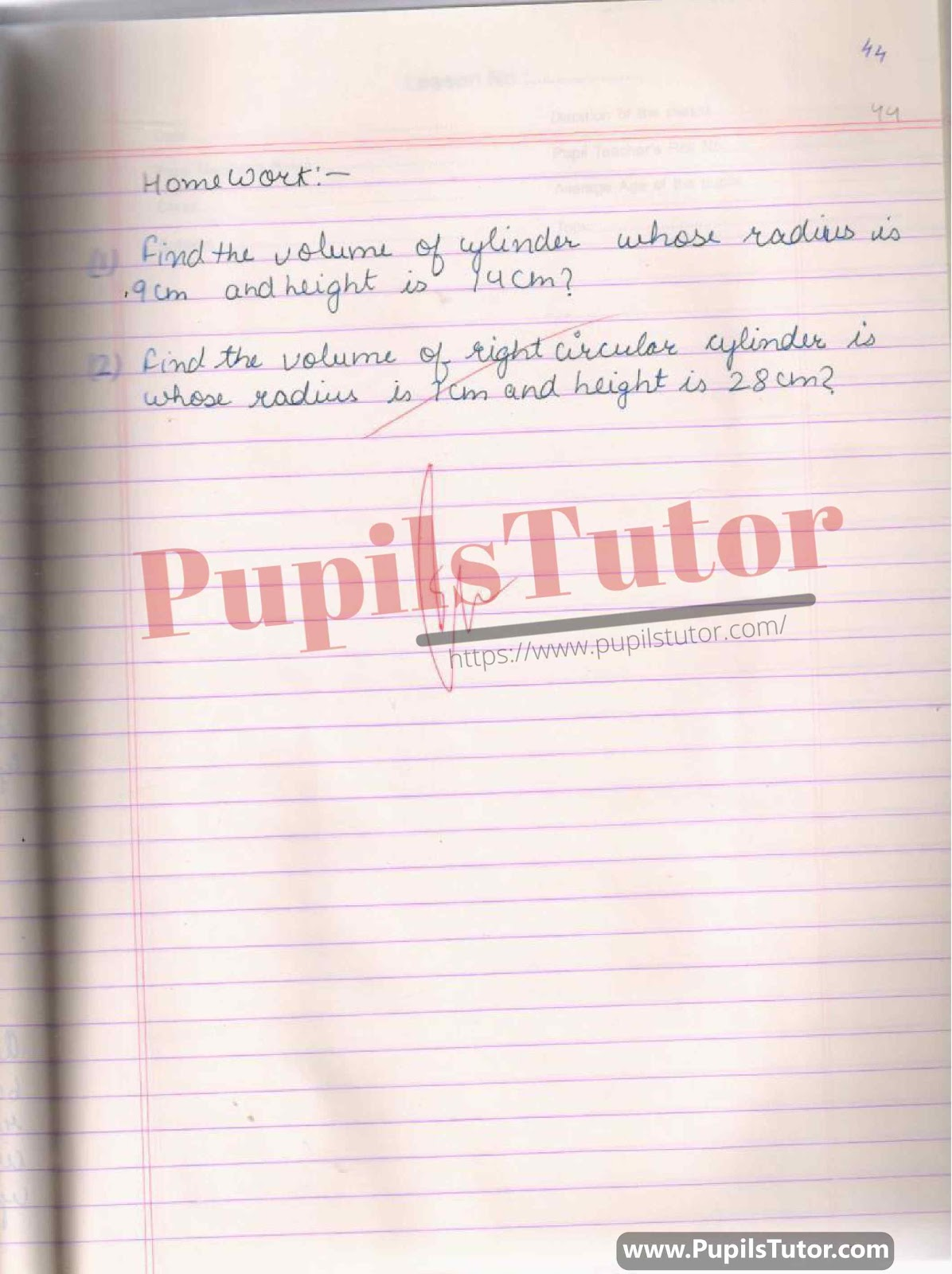You Might Also Like:

Further Reference

 B.Ed. Question Bank And Exam Notes A Complete Guide For B.ED. Entrance Examination CTET & TETs Preparation Material Teaching Aptitude and Attitude Test Book UP B.Ed Guidebook & Latest Practice Sets Book For Entrance Exam B.Ed CET Delhi University B.Ed Entrance Test Latest Practice Sets IGNOU B.Ed. Entrance Test: Previous Years Papers (Solved) Assignments And Files Lesson Planning Files And Guide

On Our Website www.PupilsTutor.Com, We Have Also Shared Lots Of Other Lesson Plans Of All Subjects On Various Teaching Skills Like Micro-Teaching, Mega Teaching, Discussion Skill, Real School Teaching And Practice Skill, Simulated And Observation Skills. You Can Also Check Them.

If You Liked This Volume Of Right Circular Cylinder Lesson Plan For Class 10 Then Please Share Our Efforts With Your Friends Also.

You Can Also Share Your Lesson Plans, Assignments, Files, Papers And Study Notes With Us To Help Other Students .

#### List Of Some More Mathematics Lesson Plans

 Profit And Loss Lesson Plan For Math Sum Of The Angles Of A Triangle Lesson Plan For Math Trigonometric Ratio Lesson Plan For Math Circle Lesson Plan For Math Properties Of Angle In Circle Lesson Plan For Math Pie Chart Lesson Plan For Math Three Dimensional Shapes Lesson Plan For Math Trigonometry Lesson Plan For Math Parts Of A Circle Lesson Plan For Math Compound Interest Lesson Plan For Math Volume Of Cone And Cylinder Lesson Plan For Math Areas Related To Circle Lesson Plan For Math Integers Lesson Plan For Math Surface Area Of Cone And Cylinder Lesson Plan For Math Statistics And Mean Lesson Plan For Math Volume Of Right Circular Cylinder Lesson Plan For Math Circular Trigonometric Functions Lesson Plan For Math 3D Shapes Lesson Plan For Math Coordinate Geometry Section Formula Lesson Plan For Math Surface Area And Volume Of Sphere Lesson Plan For Math Theorem Lesson Plan For Math Curved And Total Surface Area Of Cylinder Lesson Plan For Math Quadrilateral Lesson Plan For Math Height And Distance Lesson Plan For Math Curved And Total Surface Area Of Cone Lesson Plan For Math Coordinate Geometry Lesson Plan For Math Similar Triangle Lesson Plan For Math Polynomials Lesson Plan For Math Trigonometry Transformation Formula Lesson Plan For Math Surface Area Of Cube And Cuboid Lesson Plan For Math Algebraic Expression Lesson Plan For Math Proportions Lesson Plan For Math